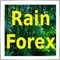# Is there such a function / operation?530

Hello!

Is there a function to tell the EA what was the initial deposit amount?

Thanks!100

Why do you want this function?530

pisceswzh wrote >>
Why do you want this function?

Hello!

I would like to set up a Money Management setting that would increase the Lots according to the percentage of profit (regarding the initial deposit). For example, if the initial deposit was 10.000 and the account has a balance of 15.000, this means a 50% profit. In this case, the Lots would be increased by 0.5. If the balance increased to, lets say, 20.000 (100% profit), the Lots would be increased another 0.5, and so on...2646

IF no trades have occurred, the AccountBalance() == theDeposit.

If trades have occurred, work back through history and add all the losses and subtract all the gains.

Easiest way might be to have an input to the EA where you say "I start with 10000"530

phy wrote >>

IF no trades have occurred, the AccountBalance() == theDeposit.

If trades have occurred, work back through history and add all the losses and subtract all the gains.

Easiest way might be to have an input to the EA where you say "I start with 10000"

Thanks!